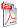Issue archive

https://doi.org/10.15255/KUI.2022.010
Published: Kem. Ind. 71 (11-12) (2022) 675–684
Paper reference number: KUI-10/2022
Paper type: Original scientific paper
Download paper:PDFDrying Process of Cement Mortar Composites Reinforced with Cellulosic Fibres: Experiment and Mathematical Modelling

A. Lachenani‬, M. Bentchikou, M. Boumahdi, S. Hanini and M. Laidi

##### Abstract

In this paper, six novel mathematical models based on semi-empirical calculus are proposed and applied to characterise the oven-drying process of cement mortar composites reinforced with cellulosic fibres (CMCRCFs). The drying experiments were carried out on four levels of oven-drying temperatures (70, 85, 105, and 120 °C), with four different cellulosic fibres content (0, 5, 10, and 20 %). Obtained results were compared to those derived by regression analysis of six most typically used mathematical drying models (Newton, Page, Page modified1, Page modified2, Handerson Pabis, and Logarithmic) in addition to six proposed models. The regression accuracy of the drying process was evaluated by the coefficient of determination (R2), low mean square error (MSE), low root mean squared error (RMSE), and mean absolute error (MAE). Additional criteria were used to ensure more validity of the selected models. The obtained values indicate a highly accurate fit of the proposed model MR9, meaning that the proposed model can clearly interpret the experimental drying data and predict the dry state of CMCRCFs.

##### Keywords

cement mortar composites, cellulosic fibres, oven drying, modelling, regression analysis